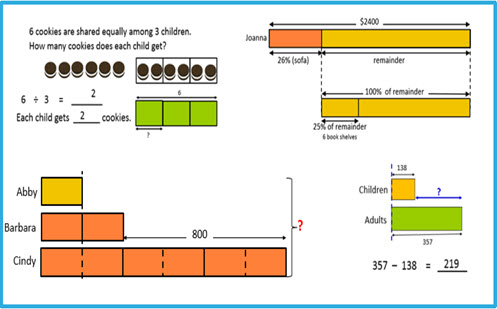Go to website

# reSolve: Bar Model Method: Introduction

This sequence of lessons introduces students to the Singapore Bar Model allowing them to visualise quantities in a problem. Students learn how to construct a model that represents the quantities in a given context. The sequence demonstrates part-whole and comparison models for whole numbers and fractions. It is recommended that these lessons are completed as a sequence. There is an overview of the Bar Model and the lessons are outlined in detail including vocabulary, curriculum links, discussion points and further activities. This sequence is part of the reSolve: Mathematics by Inquiry program.

Year level(s) Year 5
Audience Teacher
Purpose Teaching resource
Teaching strategies and pedagogical approaches Mathematics investigation
Keywords whole number, bar model, division, multiplication, subtraction, addition, fractions

## Curriculum alignment

Curriculum connections Numeracy
Strand and focus Number, Build understanding, Apply understanding
Topics Fractions, Addition and subtraction, Multiplication and division, Operating with number
AC: Mathematics content descriptions
ACMNA100 Solve problems involving multiplication of large numbers by one- or two-digit numbers using efficient mental, written strategies and appropriate digital technologies
ACMNA103 Investigate strategies to solve problems involving addition and subtraction of fractions with the same denominator
ACMNA291 Use efficient mental and written strategies and apply appropriate digital technologies to solve problems
ACMNA076 Develop efficient mental and written strategies and use appropriate digital technologies for multiplication and for division where there is no remainder
ACMNA077 Investigate equivalent fractions used in contexts
ACMNA082 Solve word problems by using number sentences involving multiplication or division where there is no remainder
ACMNA126 Solve problems involving addition and subtraction of fractions with the same or related denominators
ACMNA127 Find a simple fraction of a quantity where the result is a whole number, with and without digital technologies